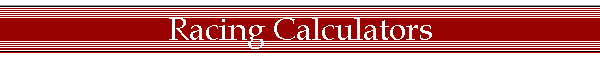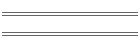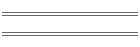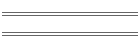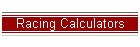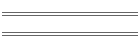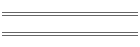### Racing Calculators:

 ¼ Mile Horsepower Calculator MPH Weight HP Enter the MPH and Weight andClick on Calculate... HP = Weight * (MPH / 234) ^3
 ¼ Mile ET & MPH Calculator #1 Weight HP ET MPH Enter the Weight and HP andClick on Calculate twice... MPH = 234 * (HP / Weight) ^ .3333ET = 1353 / MPH
 ¼ Mile ET & MPH Calculator #2 ET MPH Enter the ET or MPH andClick on Calculate... ET = 1353 / MPHMPH = 1353 / ET
 ¼ Mile ET & 1/8 Mile ET Conversions ¼ Mile ET 1/8 Mile ET Enter the ¼ Mile ET or the 1/8 Mile ET andClick on Calculate... ¼ Mile ET = (1/8 Mile ET + .22) / .6551/8 Mile ET = (¼ Mile ET * .655) - .22
 % Converter Slip Calculator RPM Tire Height Rearend Ratio Trans Ratio MPH Conv Slip% Enter the RPM, Tire Height in inches,The Rearend Gear Ratio, the MPH,the Transmission top gear ratio andClick on Calculate... Conv Slip% = [((RPM * Tire Height) / (Rearend Ratio * Trans Ratio * MPH * 336)) -1] * 100
 ¼ Mile MPH Calculator Tire Height Rearend Ratio RPM Trans Ratio Conv Slip% MPH Enter the Tire Height in inches,The Rearend Gear Ratio, the RPM,the Transmission top gear ratio,Conv Slip% andClick on Calculate... MPH = (RPM * Tire Height) / (Rearend Ratio * 336.136 * Trans Ratio * Conv Slip%)
 Optimum Rearend Ratio Calculator Tire Height MPH RPM Trans Ratio Conv Slip% Rearend Ratio Enter the Tire Height in inches,The ¼ Mile MPH, the RPM,the Transmission top gear ratioConv Slip% andClick on Calculate... Rearend Ratio = (RPM * Tire Height) / MPH * 336.136 * Trans Ratio * Conv Slip%)
 ¼ Mile RPM Calculator Tire Height Rearend Ratio MPH Trans Ratio Conv Slip% RPM Enter the Tire Height in inches,The Rearend Gear Ratio, the ¼ Mile MPH,the Transmission top gear ratioConv Slip% andClick on Calculate... RPM = (Rearend Ratio * MPH * 336.136 * Trans Ratio * Conv Slip%) / Tire Height
 Optimum Tire Height Calculator Rearend Ratio MPH RPM Trans Ratio Conv Slip% Tire Height Enter the Rearend Gear Ratio, the ¼ Mile MPH,the RPM, the Transmission top gear ratio,Conv Slip% andClick on Calculate... Tire Height = (Rearend Ratio * MPH * 336.136 * Trans Ratio * Conv Slip%) / RPM
 Engine Displacement Calculator Bore Stroke # of Cylinders Displacement Enter the Bore in inches, the stroke in inches,the number of cylinders, andClick on Calculate... Displacement = (Bore / 2) ^2 * PI * Stroke * # of cylinders
 Tire Height & Width Calculator Tire Width(mm) Aspect Ratio(1-100) Wheel Diameter(in) Tire Height(in) Tire Width(in) Enter the tire width in millimeters, the aspect ratio,the wheel diameter in inches, andClick on Calculate... Tire Height(in) = (Width(mm) / 25.4 * Aspect Ratio / 100 * 2) + Wheel diameterTire Width(in) = Width(mm) / 25.4
 Tire Width & Aspect Ratio Calculator Tire Height(in) Tire Width(in) Wheel Diameter(in) Tire Width(mm) Aspect Ratio(1-100) Enter the tire height in inches, the tire width in inches,the wheel diameter in inches, andClick on Calculate... Tire Width(mm) = Tire Width(in) * 25.4Aspect Ratio = (Tire Height - Wheel Diameter) / 2 / Tire Width * 100
 Effective & Equivalent Gear Ratio Calculator New Tire Height Old Tire Height Original Ratio Effective Ratio Equivalent Ratio Enter the New tire height, Old tire height,and the original rear gear ratio.Click on Calculate... Effective Ratio = (Old tire height / New tire height) * Original ratio Equivalent Ratio = (New tire height / Old tire height) * Original ratio
 Engine Air Capacity & Carburetor Size Calculator(normally aspirated) Displacement(in3) Max. RPM Theoretical CapacityCFM Street Carb.Size CFM Racing Carb.Size CFM Enter the engine size and maximum RPMClick on Calculate... Theoretical CFM = (Displacement * RPM) / 3456Street Carb. CFM = ((Displacement * RPM) / 3456) * 0.85Race Carb. CFM = ((Displacement * RPM) / 3456) * 1.1

Home | About Me | Photo Gallery | Track Info/Weather | Racing Calculators | Racing Links | Non-Racing Links